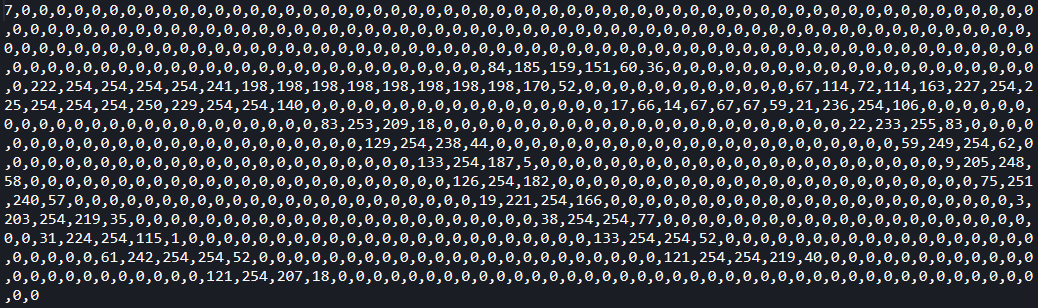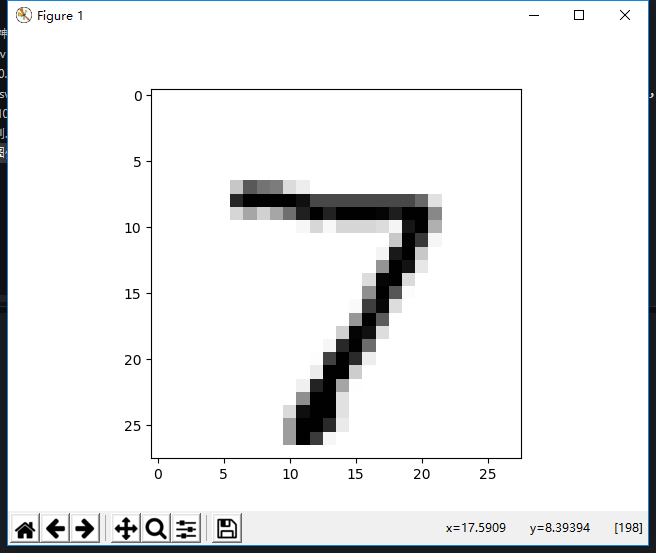训练集：http://www.pjreddie.com/media/files/mnist_train.csv

测试集：http://www.pjreddie.com/media/files/mnist_test.csv

训练集：https://raw.githubusercontent.com/makeyourownneuralnetwork/makeyourownneuralnetwork/master/mnist_dataset/mnist_train_100.csv

测试集：https://raw.githubusercontent.com/makeyourownneuralnetwork/makeyourownneuralnetwork/master/mnist_dataset/mnist_test_10.csvimport numpy as np
from matplotlib import pyplot as plt

data_file = open('mnist_test_10.csv', 'r')
data_file.close()
all_values = data_list.split(',')
image_array = np.array(all_values[1:]).astype(np.int).reshape((28, 28))
plt.imshow(image_array, cmap = 'Greys', interpolation = 'None')
plt.show()现在我们来看看Python的实现：

def loaddataset(filename):
fp = open(filename, 'r')
dataset = []
labelset = []
a = i.strip().split(',')
#对数据进行缩放
dataset.append([(int(j) / 255.0 * 0.99 + 0.01) for j in a[1:]])
labelset.append(int(a))
return np.array(dataset), np.array(labelset)

修改标签（改为[0, 0, 1, 0, 0, 0, 0, 0, 0, 0]的形式):

def adjustment_label(labelset):
new_labelset = []
for i in labelset:
new_label = [0.01, 0.01, 0.01, 0.01, 0.01, 0.01, 0.01, 0.01, 0.01, 0.01]
new_label[i] = 0.99
new_labelset.append(new_label)
return new_labelset

激活函数sigmoid使用scipy库中的expit(x)函数，网传其运行速度会更快，毕竟60000个样例，我的电脑训练一次至少要25分钟。

import scipy.special as spc
def sigmoid(z):
return spc.expit(z)

参数初始化：

def parameter_initialization(x, y, z):
value1 = np.random.normal(0.0, pow(1, -0.5), (1, y))
value2 = np.random.normal(0.0, pow(1, -0.5), (1, z))
weight1 = np.random.normal(0.0, pow(x, -0.5), (x, y))
weight2 = np.random.normal(0.0, pow(y, -0.5), (y, z))
return weight1, weight2, value1, value2

def trainning(dataset, labelset, weight1, weight2, value1, value2, x):
for i in range(len(dataset)):
print(i)
inputset = np.mat(dataset[i])
outputset = np.mat(labelset[i])
input1 = np.dot(inputset, weight1)
output2 = sigmoid(input1 - value1)
input2 = np.dot(output2, weight2)
output3 = sigmoid(input2 - value2)

a = np.multiply(output3, 1 - output3)
g = np.multiply(a, outputset - output3)
b = np.dot(g, np.transpose(weight2))
c = np.multiply(output2, 1 - output2)
e = np.multiply(b, c)

value1_change = -x * e
value2_change = -x * g
weight1_change = x * np.dot(np.transpose(inputset), e)
weight2_change = x * np.dot(np.transpose(output2), g)

value1 += value1_change
value2 += value2_change
weight1 += weight1_change
weight2 += weight2_change
return weight1, weight2, value1, value2

def testing(dataset, labelset, weight1, weight2, value1, value2):
rightcount = 0
for i in range(len(dataset)):
inputset = np.mat(dataset[i])
outputset = np.mat(labelset[i])
input1 = np.dot(inputset, weight1)
output2 = sigmoid(input1 - value1)
input2 = np.dot(output2, weight2)
output3 = sigmoid(input2 - value2)

label = np.argmax(output3)
if int(label) == int(labelset[i]):
rightcount += 1

print("真实值为%d, 预测值为%d"%(labelset[i], label))
print("正确率为%f"%(rightcount / len(dataset)))

np.argmax()返回行向量中最大值的位置

我们可以将训练好的参数用文件的形式保存，之后可以直接使用，不需要再次训练，毕竟训练一次要好久呢。我的训练好的参数文件也上传至我的资源。

正确率为0.953000
[Finished in 1005.7s]